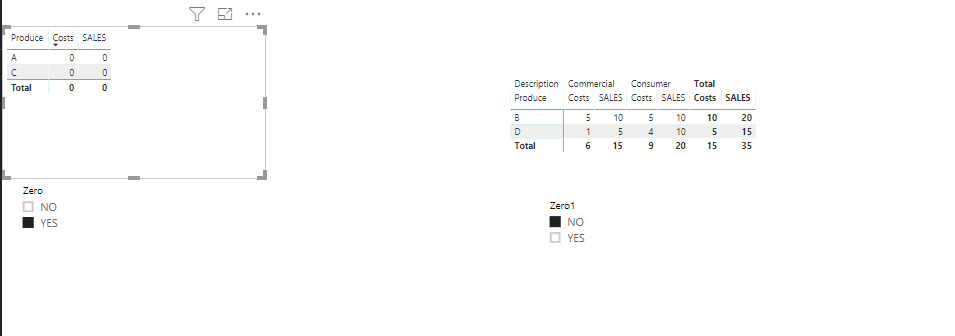cancel
Showing results for
Did you mean:Anonymous
Not applicable

## Filter Out Rows With Zeroes In All Columns.

Hi everyone,

I've been trying to create a filter, by creating a new column, to remove rows that have zeroes in all the columns in either a table format or a matrix table format. An example of data is below

Table

Product             Sales                Costs

A                          0                      0

B                         20                     10

C                          0                      0

D                         15                     5

Matrix Table

Description                                       Consumer                                  Commercial

Product                                Sales                     Costs                     Sales                   Costs

A                                           0                             0                          15                         5

B                                           20                           10                        10                         3

C                                           0                              0                         20                        10

D                                          15                            5                          10                        2

I wrote this formula for a new column:

Remove Zero Rows = if('Table Name'[2018 Q4]=0 && 'Table Name'[2017 Q4]=0),"Yes","No") and received the following error

# "The expression refers to multiple columns. Multiple columns cannot be converted to a scalar value."

2 ACCEPTED SOLUTIONSSolution Sage

You would need to create a calculated measure and use that in the visual filter to get the desired result.

Here's how the calculated measure would look like:

Zeros = CALCULATE(IF(SUM(TableName[SalesField]) = 0 && SUM(TableName[CostsField]) = 0, "Yes", "No"))Hope this helps.

Regards,

TarunCommunity Support

Hi @Anonymous ,

I created two calculated column in two tables as below.

`Zero = IF(Table1[SALES]=0 && Table1[Costs]= 0,"YES","NO")`
```Zero1 = VAR SUM1 = CALCULATE(SUM('Table1 (2)'[Costs]),ALLEXCEPT('Table1 (2)','Table1 (2)'[Produce]))
VAR SUM2 = CALCULATE(SUM('Table1 (2)'[SALES]),ALLEXCEPT('Table1 (2)','Table1 (2)'[Produce]))
RETURN
IF(SUM1=0 && SUM2=0,"YES","NO")```Reagrds,
Frank

Community Support Team _ Frank
If this post helps, then please consider Accept it as the solution to help the others find it more quickly.
6 REPLIES 6Anonymous
Not applicable

Thank you both for the solutions. Logic for formulas in DAX is really different; am still trying to get a handle on this. Thank you again.Solution Sage

What is the data field in the Values section of your table/matrix ? Is it an aggregate ? If so, you would need to use a calculated measure instead of calculated column.

Please share the screenshots or the pbix for more inputs.Anonymous
Not applicable

Thank you for the response. Data field is sales and costs and I can't post a screenshot due to client name in the visualizationSolution Sage

You would need to create a calculated measure and use that in the visual filter to get the desired result.

Here's how the calculated measure would look like:

Zeros = CALCULATE(IF(SUM(TableName[SalesField]) = 0 && SUM(TableName[CostsField]) = 0, "Yes", "No"))Hope this helps.

Regards,

TarunAnonymous
Not applicable

Thank you for the suggested solution. It didn't work as a measure; to clarify, the formula works but cannot use as a slicer because the inability to put the measure as a slicer but it works as a column; it seems that my formula logic was way off and your suggested solution works great. I just need to wrap my head around how to write proper logic in DAX, it is quite different from what I'm used to in Excel and other ETL and visualization tools.

Before I mark your answer as solution, how would this be accommodate for the matrix array? Let's says Sales and Costs are already rows in the original data but is then pivoted in a matrix table as columns. So in my second matrix tables, those sales and costs are rows and data fields are Consumer and Commercial.Community Support

Hi @Anonymous ,

I created two calculated column in two tables as below.

`Zero = IF(Table1[SALES]=0 && Table1[Costs]= 0,"YES","NO")`
```Zero1 = VAR SUM1 = CALCULATE(SUM('Table1 (2)'[Costs]),ALLEXCEPT('Table1 (2)','Table1 (2)'[Produce]))
VAR SUM2 = CALCULATE(SUM('Table1 (2)'[SALES]),ALLEXCEPT('Table1 (2)','Table1 (2)'[Produce]))
RETURN
IF(SUM1=0 && SUM2=0,"YES","NO")```Reagrds,
Frank

Community Support Team _ Frank
If this post helps, then please consider Accept it as the solution to help the others find it more quickly.